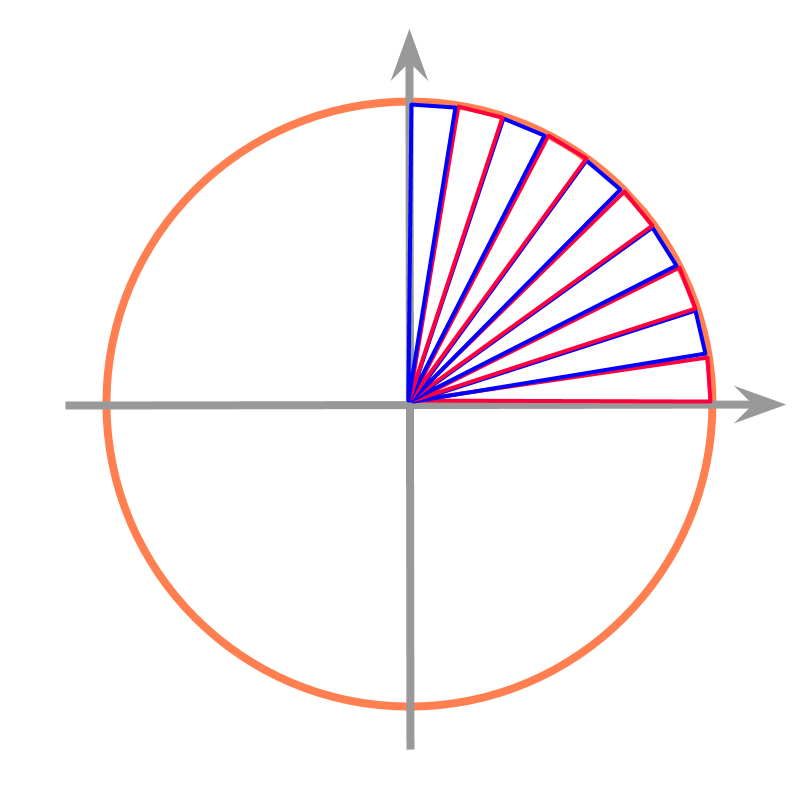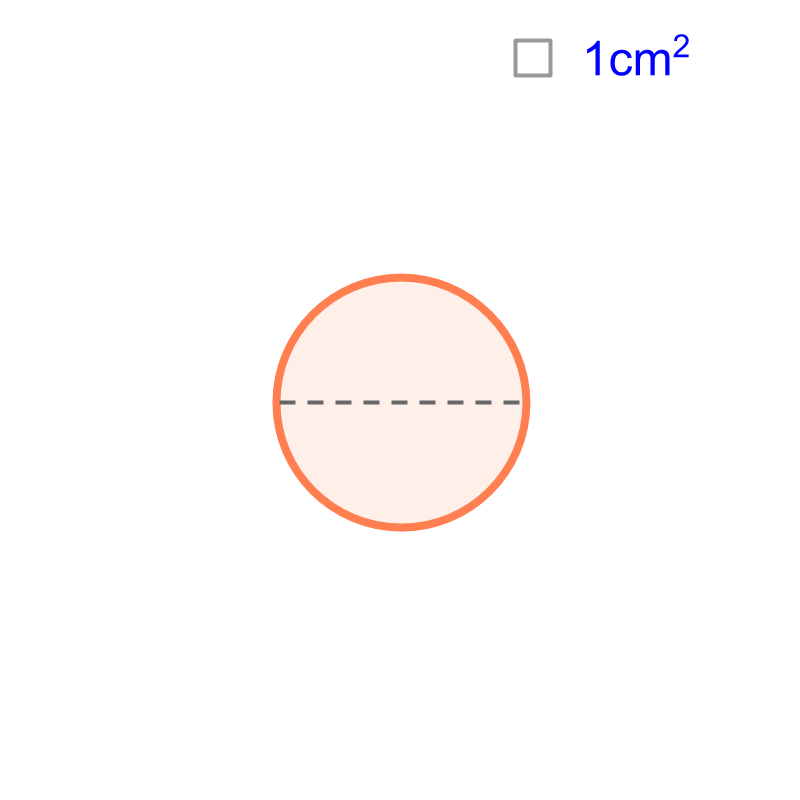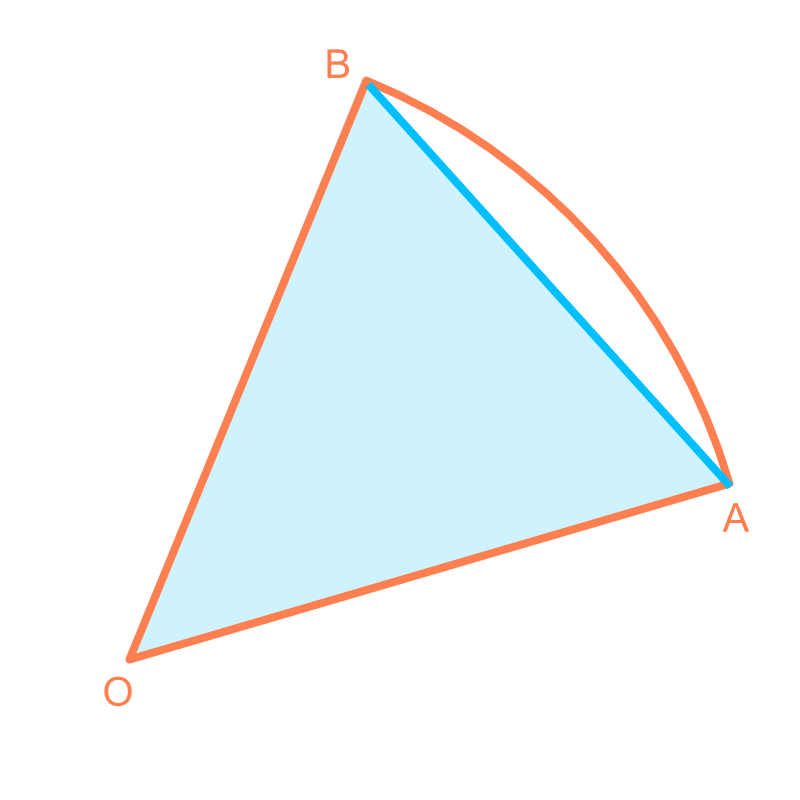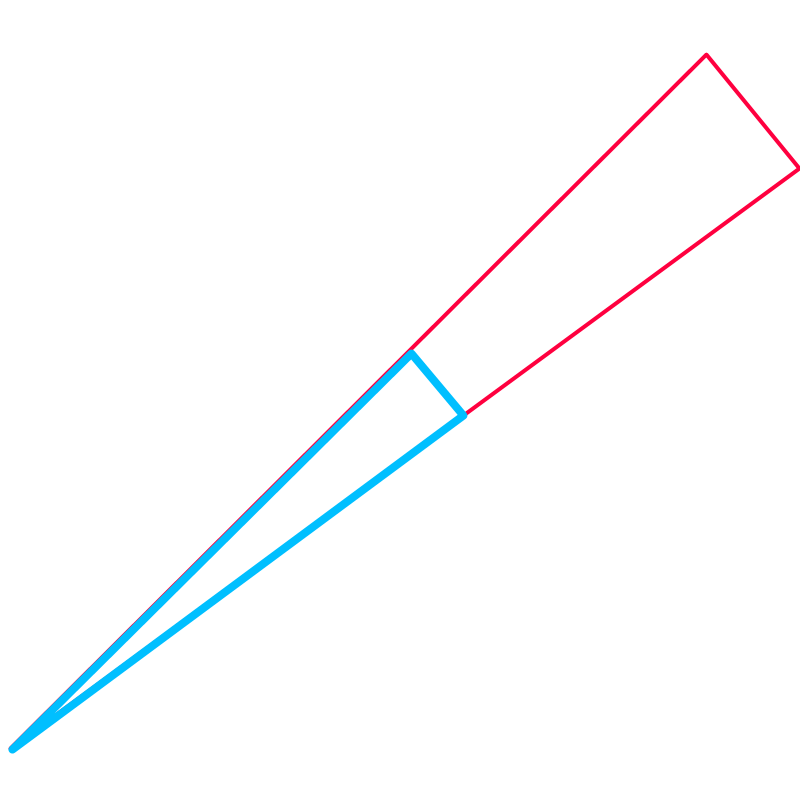maths > mensuration-high

Circumference of a Circle

what you'll learn...

Overview

Circumference of a Circle: : Circumference of a circle of $1$$1$ cm diameter is computed to be a decimal value that does not end or repeat. It is calculated to be $3.14159\cdots$$3.14159 \cdots$ by approximating the circle to infinitesimally small triangular pieces.Circumference $=2\pi r$$= 2 \pi r$ and $=\pi d$$= \pi d$ $r$$r$ is the radius of the circle

$d$$d$ is the diameter of the ci

recap

We learned about measurement of objects in real-life, or a line segment of arbitrary length. Some of the learnings are:

•  The length of an object can be an integer value in the unit of measurement. eg: $2$$2$ cm

•  The length of an object need not be exactly an integer value in the unit of measurement. It can be given in smaller unit of length. eg: Length of a pencil is $2cm5mm$$2 c m 5 m m$ or $=25mm$$= 25 m m$ or $=2.5$$= 2.5$ cm

•  The length of an object can be a decimal number in which the decimals do not repeat or do not end. The practical measure of the length is limited by the accuracy of the instrument used in measuring. eg: Length of a pencil is $2.512847\cdots$$2.512847 \cdots$ cm.

Similarly, the circumference of a circle of $1$$1$ cm diameter is a decimal number in which the decimals do not repeat or do not end. That number is approximately $3.14$$3.14$ cm or $\frac{22}{7}$$\frac{22}{7}$ cm.The circumference of a circle of diameter $1$$1$ cm is
$\pi$$\pi$ cm
=approximately $\frac{22}{7}$$\frac{22}{7}$ cm
= approximately $3.14$$3.14$ cm

Circumference of a circle of diameter $1$$1$ cm is represented as $\pi$$\pi$. For a circle of diameter $d$$d$ cm or radius $r$$r$ cm, the circumference is $\pi d$$\pi d$ or $2\pi r$$2 \pi r$ cm.

The circumference of a circle of $7$$7$ cm radius is "$44$$44$ cm"
Approximately $\pi =\frac{22}{7}$$\pi = \frac{22}{7}$

The above was at beginner level understanding. Now, let us learn how to calculate the circumference of a circle.

calculatingConsider the circle given in the figure. The diameter of the circle is $7$$7$ cm. We can visualize the circle as a combination of isosceles-triangles to find sum of the bases as approximate circumference.Consider finding circumference of a circle spanned with triangles as illustrated in the figure.The base of a triangle $\overline{AB}$$\overline{A B}$ is an approximation to the corresponding arc on the circle $AB$$A B$. The accuracy of the calculated circumference value can be increased if the triangle is made thinner (angle between equal sides is smaller).

solution for unit circle

The circumference of a circle is calculated by one of many methods involving equivalence and approximation. For now, consider the diameter to be $1$$1$ cm. The circumference of the circle is calculated to be $3.14159\cdots$$3.14159 \cdots$ by approximating the circle to infinitesimally small triangular pieces.

Remember we studied that length of a line-segment can be measured finer and finer to an accurate value -- a decimal number that does not terminate and does not repeat. Similarly, the circumference of a circle is a decimal number that does not terminate and does not repeat.

The circumference of a circle of diameter $1$$1$ cm is commonly approximated to a value $\frac{22}{7}$$\frac{22}{7}$ or $3.14$$3.14$.

To represent the accurate decimal value, the number is represented with the symbol $\pi$$\pi$. It is an irrational number (a decimal number that does not end or repeat).

solution for any circleThe circumference of a circle with diameter $1$$1$ cm is computed as a value represented with $\pi$$\pi$.

What is the circumference of a circle with diameter $2$$2$ cm or $20$$20$ cm? Will there be more numbers defined like $\pi$$\pi$?

The question is answered with some geometrical calculations. Consider one piece of the triangle that was used to calculate circumference of a circle of diameter $1$$1$ cm. The triangle is shown in blue color in the figure.

The corresponding triangle for a circle of $d$$d$ cm is shown in red. Note that the triangles overlap and the blue triangle is shown on top of the red one.

The corresponding angles of these two triangles are equal and thus the triangles are similar.

The property of similar triangles is that, the ratio of the sides are constant.

The isosceles sides of blue triangle are $1/2$$1 / 2$ cm and the base is $x$$x$.
The isosceles sides of red triangles are $d/2$$d / 2$ cm and the base is $y$$y$.

As per property of similar triangles,
$1/2÷1/d=x÷y$$1 / 2 \div 1 / d = x \div y$
$\to y=d×x$$\to y = d \times x$
This means, the base of the triangles from larger circle are $d$$d$ times the base of triangles from smaller circle.
This implies that, the circumference of larger circle of $d$$d$ cm diameter is $d$$d$ times the circumference of the smaller circle.
The circumference of the smaller circle of $1$$1$ cm diameter is $\pi$$\pi$.

This implies that circumference of the larger circle is $d×\pi$$d \times \pi$

Summarizing the derivation

Circumference of a circle of $1$$1$ cm diameter is computed to be a decimal value that does not end or repeat. That accurate value is represented by the symbol $\pi$$\pi$ and approximate value of that is $\frac{22}{7}$$\frac{22}{7}$ and $3.14$$3.14$.

Circumference of a circle of $d$$d$ cm diameter is computed to be $d$$d$ times the circumference of the circle of $1$$1$ cm diameter.

Thus the generic formula for the circumference of a circle is $\pi d$$\pi d$ or $2\pi r$$2 \pi r$.

What is the circumference of a circle of $\frac{1}{\pi }$$\frac{1}{\pi}$cm diameter?
The answer is "$1$$1$ cm"

summary

Circumference of a Circle: : Circumference of a circle of $1$$1$ cm diameter is computed to be a decimal value that does not end or repeat. It is calculated to be $3.14159\cdots$$3.14159 \cdots$ by approximating the circle to infinitesimally small triangular pieces.Circumference $=2\pi r$$= 2 \pi r$ and $=\pi d$$= \pi d$ $r$$r$ is the radius of the circle

$d$$d$ is the diameter of the circle

Outline